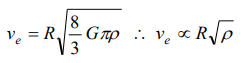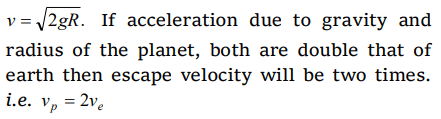## Gravitation Questions and Answers Part-16

1. How many times is escape velocity $v_{e}$ , of orbital velocity $v_{0}$ for a satellite revolving near earth
a) $\sqrt{2}$  times
b) 2 times
c) 3 times
d) 4 times

Explanation: $\sqrt{2}$  times

2. Escape velocity on earth is 11.2 km/s. What would be the escape velocity on a planet whose mass is 1000 times and radius is 10 times that of earth
a) 112 km/s
b) 11.2 km/s
c) 1.12 km/s
d) 3.7 km/s

Explanation:3. If the radius of a planet is R and its density is ρ , the escape velocity from its surface will be
a) $v_{e}\propto \rho R$
b) $v_{e}\propto \sqrt{\rho }R$
c) $v_{e}\propto \frac{\sqrt{\rho}}{R}$
d) $v_{e}\propto \frac{1}{\sqrt{\rho }R}$

Explanation:4. Escape velocity on the earth
a) Is less than that on the moon
b) Depends upon the mass of the body
c) Depends upon the direction of projection
d) Depends upon the height from which it is projected

Explanation:5. If acceleration due to gravity on the surface of a planet is two times that on surface of earth and its radius is double that of earth. Then escape velocity from the surface of that planet in comparison to earth will be
a) 2 ve
b) 3 ve
c) 4 ve
d) None of these

Explanation:6. The escape velocity of a rocket launched from the surface of the earth
a) Does not depend on the mass of the rocket
b) Does not depend on the mass of the earth
c) Depends on the mass of the planet towards which it is moving
d) Depends on the mass of the rocket

Explanation: Does not depend on the mass of the rocket

7. The ratio of the radii of planets A and B is $k_{1}$ and ratio of acceleration due to gravity on them is $k_{2}$ . The ratio of escape velocities from them will be
a) $k_{1}k_{2}$
b) $\sqrt{k_{1}k_{2}}$
c) $\sqrt{\frac{k_{1}}{k_{2}}}$
d) $\sqrt{\frac{k_{2}}{k_{1}}}$

Explanation:8. A mass of $6\times 10^{24}kg$   is to be compressed in a sphere in such a way that the escape velocity from the sphere is $3\times 10^{8} m\diagup s$   . Radius of the sphere should be (G = $66.7\times 10^{-11}N - m^{2}\diagup kg^{2}$     )
a) 9 km
b) 9 m
c) 9 cm
d) 9 mm

Explanation:9. Escape velocity on the surface of earth is 11.2km/s . Escape velocity from a planet whose mass is the same as that of earth and radius 1/4 that of earth is
a) 2.8 km/s
b) 15.6 km/s
c) 22.4 km/s
d) 44.8 km/s10. The radius of a planet is $\frac{1}{4}$ of earth’s radius and its acceleration due to gravity is double that of earth’s acceleration due to gravity. How many times will the escape velocity at the planet’s surface be as compared to its value on earth’s surface
a) $\frac{1}{\sqrt{2}}$
b) ${\sqrt{2}}$
c) ${2\sqrt{2}}$